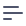# Execution Environment

Cube.js Schema Compiler uses Node.js VM to execute schema compiler code. It gives required flexibility allowing transpiling schema files before they get executed, storing schemas in external databases and executing untrusted code in a safe manner. Cube.js Schema JavaScript is standard JavaScript supported by Node.js starting in version 8 with the following exceptions.

## Require

Being executed in VM data schema, JavaScript code doesn't have access to Node.js require directly. Instead require() is implemented by Schema Compiler to provide access to other data schema files and to regular Node.js modules. Besides that, the data schema require() can resolve Cube.js packages such as Funnels unlike standard Node.js require().

## Node.js globals (process.env, console.log and others)

Data schema JavaScript code doesn't have access to any standard Node.js globals like process or console. In order to access process.env, utility functions can be added outside the schema/ directory:

tablePrefix.js:

exports.tableSchema = () => process.env.TABLE_SCHEMA;


schema/Users.js:

import { tableSchema } from '../tablePrefix';

cube(Users, {
sql: SELECT * FROM ${tableSchema()}.users, // ... });  ## console.log Data schema cannot access console.log due to a separate VM instance that runs it. Suppose you find yourself writing complex logic for SQL generation that depends on a lot of external input. In that case, you probably want to introduce a helper service outside of schema directory that you can debug as usual Node.js code. ## Cube.js globals (cube and others) Cube.js defines cube(), context() and asyncModule() global variable functions in order to provide API for schema configuration which aren't normally accessible outside of Cube.js schema. ## Import / Export Data schema JavaScript files are transpiled to convert ES6 import and export expressions to corresponding Node.js calls. In fact import is routed to Require method. export can be used to define named exports as well as default ones: constants.js: export const TEST_USER_IDS = [1, 2, 3, 4, 5];  usersSql.js: export default (usersTable) => select * from${usersTable};


Later, you can import into the cube, wherever needed:

Users.js:

// in Users.js
import { TEST_USER_IDS } from './constants';

cube(Users, {
sql: usersSql(users),
measures: {
/* ... */
},

dimensions: {
/* ... */
},

segments: {
excludeTestUsers: {
sql: ${CUBE}.id NOT IN (${TEST_USER_IDS.join(', ')}),
},
},
});


## asyncModule

Schemas can be externally stored and retrieved through an asynchronous operation using the asyncModule(). For more information, consult the Dynamic Schema Creation page.

## Context symbols transpile

Cube.js uses a custom transpiler to optimize boilerplate code around referencing cubes and cube members. There are reserved property names inside cube definition that undergo reference resolve transpiling process:

• sql
• measures
• dimensions
• segments
• timeDimension
• drillMembers
• contextMembers

Each of these properties inside cube and context definitions are transpiled to functions with resolved arguments. For example:

cube(Users, {
// ...

measures: {
count: {
type: count,
},

ratio: {
sql: sum(${CUBE}.amount) /${count},
type: number,
},
},
});


is transpiled to:

cube(Users, {
// ...

measures: {
count: {
type: count,
},

ratio: {
sql: (CUBE, count) => sum(${CUBE}.amount) /${count},
type: number,
},
},
});


So for example if you want to pass the definition of ratio outside of the cube, you would define it as:

const measureRatioDefinition = {
sql: (CUBE, count) => sum(${CUBE}.amount) /${count},
type: number,
};

cube(Users, {
// ...

measures: {
count: {
type: count,
},

ratio: measureRatioDefinition,
},
});